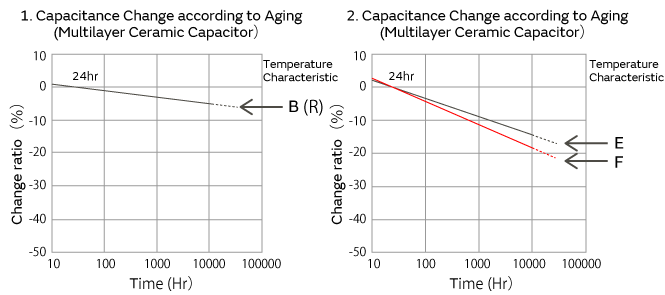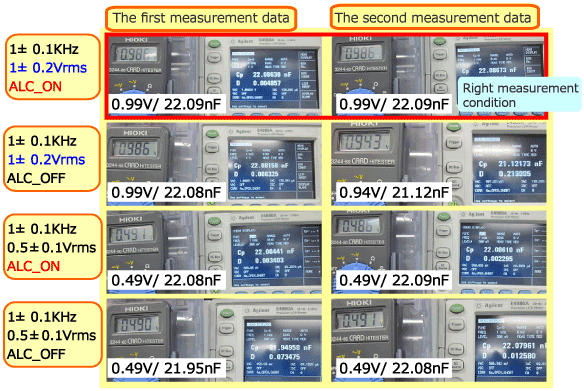# Ceramic CapacitorPrecautions for measuring the capacitance of chip multilayer ceramic capacitors

## Precautions for measuring capacitance

Do any of the following problems occur when measuring the capacitance of chip multilayer ceramic capacitors (hereinafter, "MLCC")?

Let's resolve these problems!!

• *Click to move to each item.The low-capacity MLCC of the temperature compensation type is larger or smaller than the nominal value when the capacitance is measured.The high-capacity MLCC of the high dielectric constant type capacitor is smaller than the nominal value when the capacitance is measured.

## Details for measurement of temperature compensating type capacitor

The low-capacity MLCC of the temperature compensation type is larger or smaller than the nominal value when the capacitance is measured.

The process is explained using the following steps.

### 1)What is the test fixture zero point correction?

1. The MLCC is measured as shown in the figure below, but the following issues occurred, and the actual MLCC capacitance cannot be accurately measured.
• There is series resistance and series inductance in the measuring cable
• There is stray capacitance in the measuring terminal, which interposes the MLCC
Therefore, the action, which cancels out each parameter when the MLCC being measured is not present, is called the "Test Fixture Zero Point Correction."
This corresponds to the OPEN correction and SHORT correction actions taken before the measurement.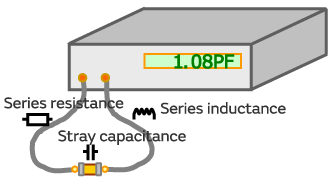2. The OPEN correction cancels out the stray capacitance of the measuring terminal which interposes the MLCC while the SHORT correction cancels out the series resistance and inductance in the measuring cable.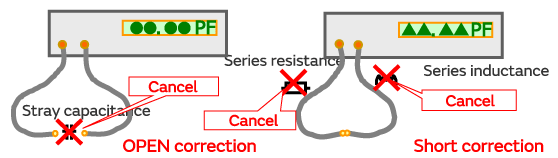3. After performing this OPEN correction and SHORT correction, you can ensure the accuracy of the MLCC measurement.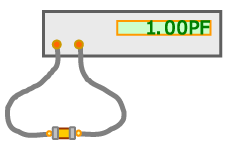### 2)The distance between the terminals and the capacitance measurement value during OPEN adjustment

The capacitance of a 1 pF MLCC was measured while changing the distance between the terminals of the test fixture during the OPEN correction.
As a result, when the distance between the terminals during the OPEN correction is greater than the L dimension of the MLCC being measured, the capacitance increases, and decreases when it is smaller.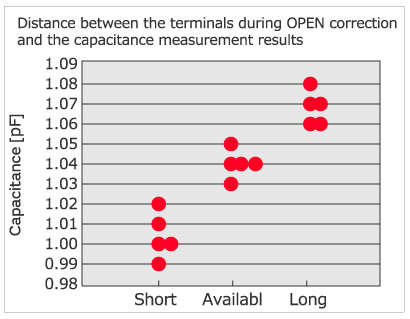#### Measurement conditions

Test sample
GRM0334C1H1R0B
Measuring instrument
HP4278A
Measuring jig
HP TEST FIXTURE16034E (insertion type)
Conditions
1±0.1MHz/1±0.2Vrms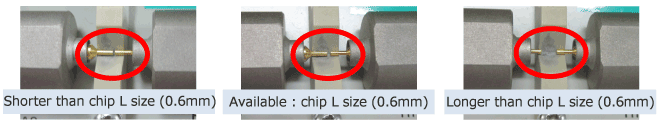### 3)The reason why the capacitance measurement value changed

#### Why does the capacitance change depending on the distance between the terminals during OPEN correction?

Capacitance occurs when there is an insulator between two pieces of metal.
The air is also an insulator, so capacitance occurs between the measuring terminals.

The capacitance increases as the distance between the two pieces of metal decreases.
Therefore, as the distance between the terminals gets smaller, the capacitance gets larger.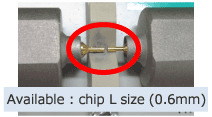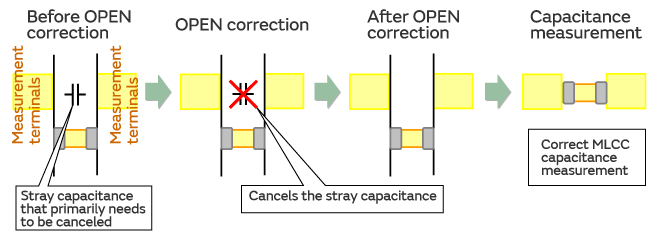#### Distance between the terminals during OPEN correction and the capacitance measurement results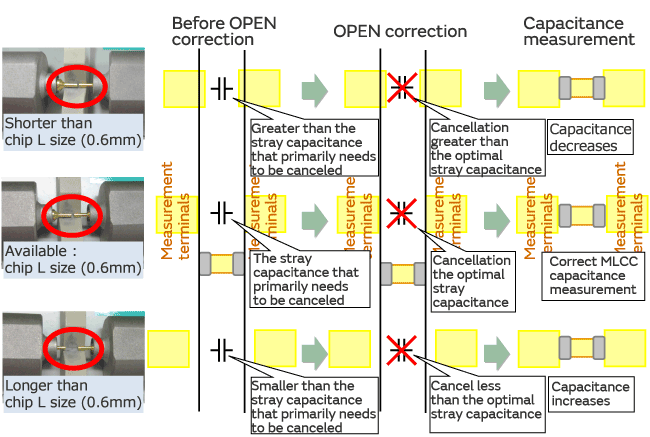### 4)Precautions during measurement

#### Key points during OPEN correction

Set the distance between the fixture terminals during OPEN correction to the same value as the L dimension of the chip being measured.

If OPEN correction is performed when the distance between the fixture terminals is long, the fixture stray capacitance during correction will be smaller than the capacitance during the actual measurement.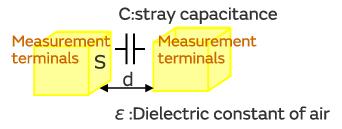Ｃ＝ε・Ｓ／ｄ ・・・・・ Because "d" increases in formula (1)

stray capacitance (capacitance)
ε
dielectric constant
electrode surface area
distance between terminals (distance between electrodes)

If the zero point correction is performed when the distance between the fixture terminals differs from the dimensions of the MLCC being measured, the stray capacitance of the fixture itself will not be accurately corrected to zero.

When the distance between the terminals during OPEN correction is smaller than the L dimension of the MLCC, the stray capacitance of the fixture is corrected to the zero point at a distance greater than reality, so the post-correction measurement result will decrease.

Conversely, if the distance between the terminals is set to greater than the L dimension of the MLCC, the post-correction measurement result will increase.
The variation in the distance between the terminals during OPEN correction is greater when using a tweezer-type of fixture (Ex. Agilent16334) than it is when using an insertion-type of fixture (Ex. Agilent16034).

Compared to the insertion-type of fixture, the tweezer-type has a measuring terminal tip with a larger surface area (S in formula 1), so the fluctuation in the capacitance measurement value increases according to the difference in the distance between the terminals.

## Details for measurement of high dielectric constant type capacitor

The high-capacity MLCC of the high dielectric constant type capacitor is smaller than the nominal value when the capacitance is measured.

The process is explained using the following steps.

### 1)Example of a high capacity MLCC measurement

Measured electrostatic capacity of high-capacity and non high-capacity in the state of ALC (Automatic Level Control ) ON/OFF ,the results are as follows.

#### Measurement conditions

Test sample
GRM188R60J106K/ GRM188B11H103K
Measuring instrument
Agilent E4980A
Measuring jig
Agilent TEST FIXTURE16334 (tweezer-type)
Conditions
GRM188R60J106K ；1±0.1KHz/0.5±0.1Vrms
GRM188B11H103K； 1±0.1KHz/1.0±0.2Vrms

⇒When measuring the high capacity capacitance, the measurement result was smaller compared to when the ALC was turned ON only when the ALC was OFF.

#### Capacitance measurement results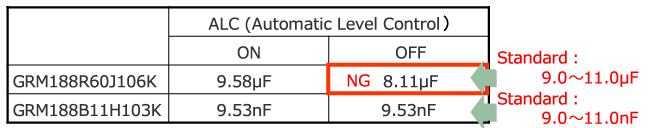### 2)Reason why the capacitance falls below the measured value

The results of measuring the voltage with a tester in each case are as follows.
In a high capacity MLCC measurement, the measurement voltage does not meet the standard conditions when the ALC is OFF.

#### Measurement conditions：1±0.1KHz/0.5±0.1Vrms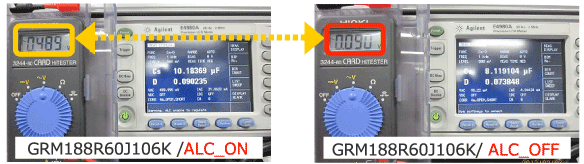#### Measurement conditions：1±0.1KHz/1.0±0.2Vrms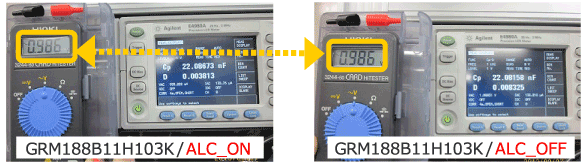#### Why does the measurement voltage Vc decrease when the capacitance increases?

The capacitance C is expressed as

•When the capacitance C increases, Zc decreases.
In addition, the measurement voltage Vc in the measurement circuit can be expressed as

•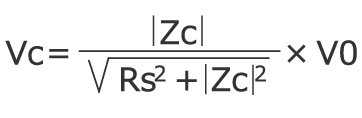Therefore, the measurement voltage Vc decreases when Zc drops.

•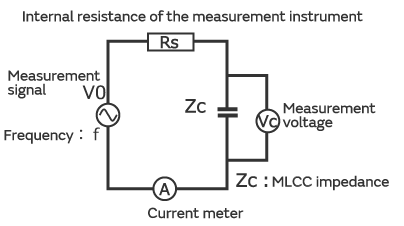#### Why does the capacitance measurement result decrease when the measurement voltage Vc decreases?

The MLCC capacitance changes based on the ambient temperature and the applied voltage.
The AC voltage characteristics of the MLCC at measurement conditions of 20°C and 1 KHz are shown in the figure on the right.
The capacitance changes when the AC voltage changes, and when the applied voltage is less than 0.5 Vrms, the capacitance also decreases.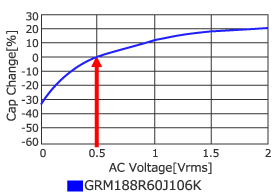### 3)Precautions during measurement

#### When the capacitance drops, use the tester to measure the measurement voltage.

If the measurement voltage is less than the standard measurement voltage, perform the following steps

1. Turn the ALC ON
2. Switch to a measuring instrument which exhibits the standard measurement voltage.

To measure the measurement voltage, apply the tester to each of the measuring terminals during the MLCC measurement as shown in the photograph below.## About aging of high dielectric constant type capacitor

The main component of high dielectric constant type capacitor is barium titanate (BaTiO3).
This series of capacitors with static capacity over time and become smaller phenomenon. This phenomenon is called the aging characteristics of electrostatic capacity.

BaTiO3 system is shown in Figure 1,it is such a cubic when the temperature above the Curie point.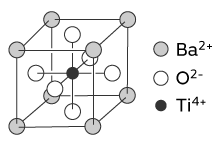Figure 1:
Crystal structure of BaTiO3 capacitor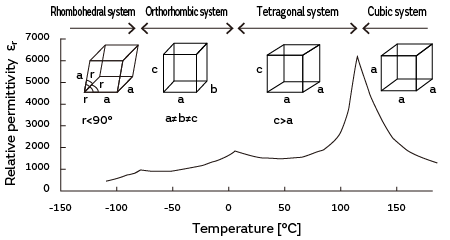Figure 2:
Crystalline structure with temperature and changes in dielectric rate (BaTiO3)

When the BaTiO3 ceramic capacitor is heated above the Curie point, the crystal structure is converted from tetragonal to cubic. When the temperature is below the Curie point, the crystal structure is converted from cubic to tetragonal.（Figure 2）
In a word, when the fine structure of the crystal is heated to the temperature above the Curie point, it will recover to the initial state, and the aging will start again.

Due to the reduced electrostatic capacity of aging, the heating process in the installation works of your company can be recovered.